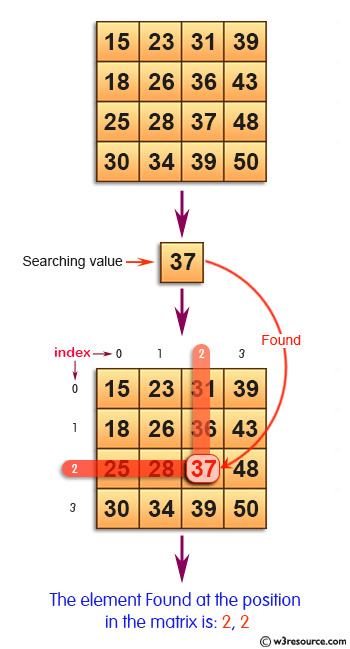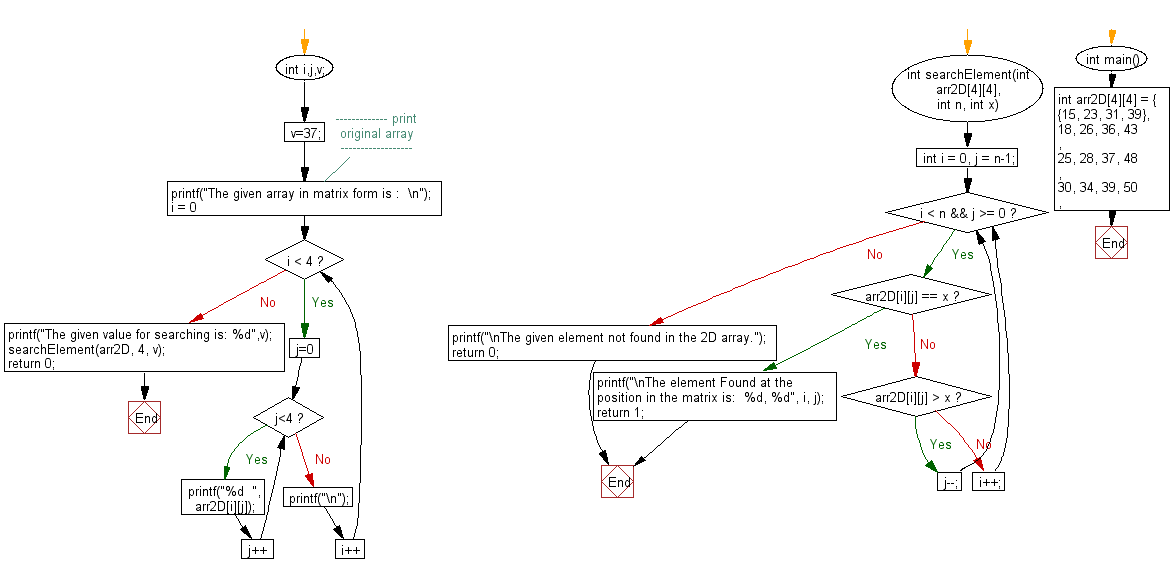﻿ C exercises: Search an element in a row wise and column wise sorted matrix - w3resource# C Exercises: Search an element in a row wise and column wise sorted matrix

## C Array: Exercise-67 with Solution

Write a program in C to search an element in a row wise and column wise sorted matrix.

Pictorial Presentation:Sample Solution:

C Code:

``````#include <stdio.h>
int searchElement(int arr2D, int n, int x)
{
int i = 0, j = n-1;
while ( i < n && j >= 0 )
{
if ( arr2D[i][j] == x )
{
printf("\nThe element Found at the position in the matrix is:  %d, %d", i, j);
return 1;
}
if ( arr2D[i][j] < x )
j--;
else
i++;
}
return 0;
}

int main()
{
int arr2D = { {15, 23, 31, 39},
{18, 26, 36, 43},
{25, 28, 37, 48},
{30, 34, 39, 50},
};
int i,j,v;
v=37;
//------------- print original array ------------------
printf("The given array in matrix form is :  \n");
for(i = 0; i < 4; i++)
{
for (j=0;j<4;j++)
{
printf("%d  ", arr2D[i][j]);
}
printf("\n");
}
//------------------------------------------------------
printf("The given value for searching is: %d",v);
searchElement(arr2D, 4, v);
return 0;
}
```
```

Sample Output:

```The given array in matrix form is :
15  23  31  39
18  26  36  43
25  28  37  48
30  34  39  50
The given value for searching is: 37
The element Found at the position in the matrix is:  2, 2
```

Flowchart:C Programming Code Editor:

Improve this sample solution and post your code through Disqus.

What is the difficulty level of this exercise?

﻿

## C Programming: Tips of the Day

Where is the C auto keyword used?

auto is a modifier like static. It defines the storage class of a variable. However, since the default for local variables is auto, you don't normally need to manually specify it.

Ref : https://bit.ly/3yzwC9r